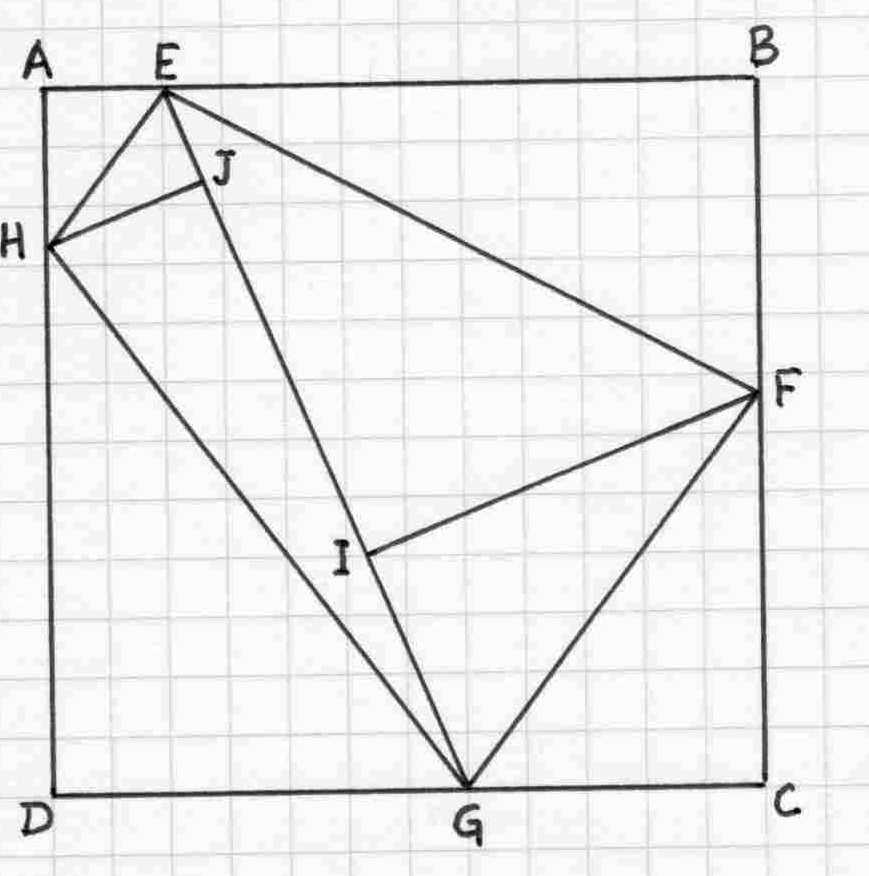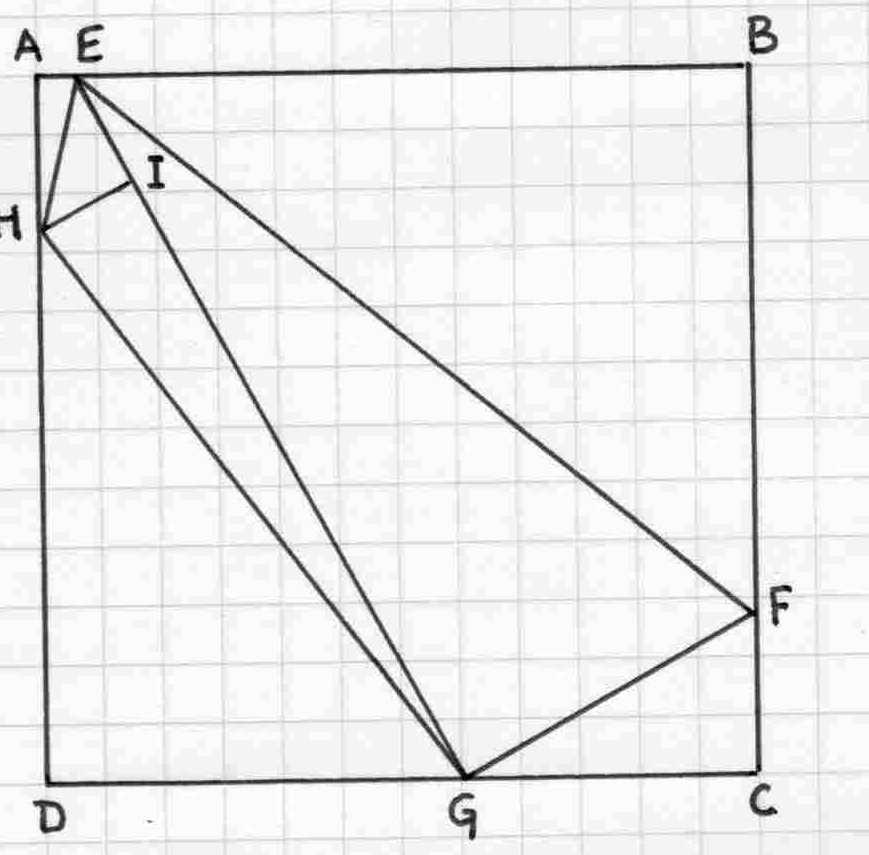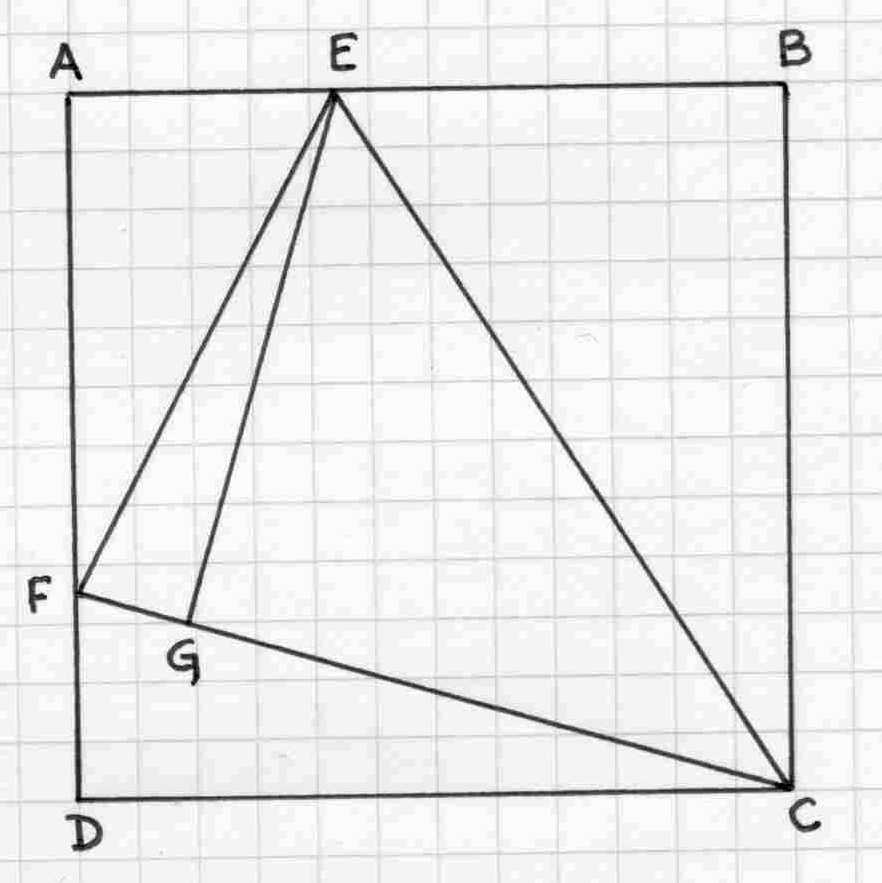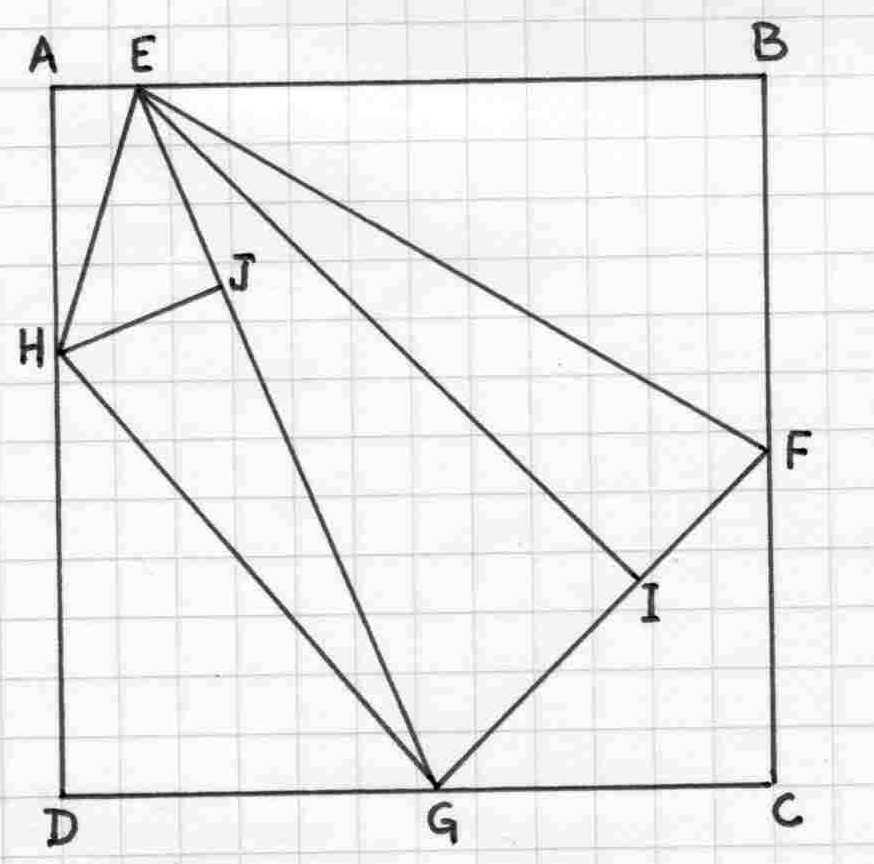## Right Triangles in a Square

1. Find the smallest square which can be divided into unique (non-identical) right triangles, such that all sides of the triangles are integers.
2. Find the smallest square which can be divided into non-similar right triangles, such that all sides of the triangles are integers.
3. Find the smallest square which can be divided into 8 non-similar right triangles, such that all sides of the triangles are integers.
Source: Denis Borris.
Solutions were received from Denis Borris and Philippe Fondanaiche.  Philippe's solutions follow.

Question 1
Let a square ABCD.
The first natural method to divide this square in right triangles is to try to find:
1) a point E on AB such that ADE and BCE are right triangles and the triangle CED is split in two right
triangles with the height drawn from C (or from D). Let AB=n and AE=a with a and n integers a<>n/2.
We have to find a and n such that a^2+n^2 is a square and (n-a)^2+n^2 is also a square.
There is no solution for n<5000 and probably whatever n.
or
2) a point M within the square such that MA,MB,MC and MD are integers. If this point exists, it is easy
to divide the square in 4 pairs of identical right triangles and to subdivide one triangle of each pair into two
unique right triangles.
Such a point M does not exist....

So we have considered many other approaches. Only one of them provides a significant number of possible
solutions and consists of finding 4 points E,F,G,H respectively on AB,BC,CD and DE such that the triangles
AEH, BEF, CFG and DGH are Pythagorean and the diagonal EG or HF is an integer (see the diagram hereafter)With a square of side equal to 468, we obtain 8 eight right triangles with the following measures:
The sides of the right triangles are a1<a2<a3.
If the ratios a1/a2 are the same, the corresponding triangles are similar.

triangles               a1          a2          a3          a1/a2
AEH                    78         104        130          0,75
BEF                   208         390        442          0,5333..
CFG                   195         260        325          0,6
DGH                   273         364        455          0,75
EFI                     280         342        442          0,81871...
FGI                    165          280        325          0,589285...
EHJ                     66          112        130          0,589285...
GHJ                   112          441        455          0,253968..

Question 2

With the same approach as above but with the constraint that all the ratios a1/a2 are different, we get
a square of length n=3060 which can be divided into 7 right triangles as EGF is already a right triangle.triangles               a1          a2          a3            a1/a2
AEH                  153         680         697          0,225
BEF                  2380       2907       3757         0,81871..
CFG                   680       1275        1445         0,5333..
DGH                 1785       2380        2975         0,75
EHI                    455         528          697         0,861742...
EFG                  1445       3468        3757        0,41666..
GHI                     455       2940        2975        0,154761..

We can take the opportunity to look for a square divided into a minimum of right triangles.
In this case, the pattern is defined hereafter with a point E on AB and a point F on AD. We succeed
to divide the square into only 5 right triangles. The side of the square is n=9000.triangles               a1          a2          a3             a1/a2
AEF                  3400       6375       7225          0,53333...
BEC                  5600       9000      10600         0,62222...
CDF                  2625       9000        9375         0,291666..
EFG                  1479       7072       7225          0,2091346...
CFG                  7072       7896       10600         0,895643...

Question 3

We use again the same approach as in Q1 and Q2 and we get a square of side equal to 3276
divided into 8 non-similar right triangles with the following diagram and measures:triangles               a1          a2          a3           a1/a2
AEH                   364        1248      1300        0,291666..
BEF                  1716        2912      3380        0,589285..
CFG                  1547        1560      2197        0,991666...
DGH                  1729        2028      2665        0,852564....
EHJ                    816         1012     1300         0,806324...
EFI                     832         3276     3380         0,253968...
EGI                   1365         3276     3549         0,416666...
GHJ                   816          2537     2665         0,321639....

Mail to Ken